# Irr with dates with large transactions listsHi anaplan community,

We have a challenge using irr dates with large transactions lists.

Challenge :

- transactions are loaded from a large flat file list of transactions

- irr dates functions calculates number of days di as the difference between the first transaction and the transaction date.

- when the transactions are loaded, the first transactions in the list isnt the first transaction date (e.g. 1st transaction may be a date in 2023).

- the flat file it self wont be ordered by ascending dates and can have multiple cf on a same date with both cash out and in

- transaction list is targeted to be production & numbered list

Has anyone encountered a similar challenge and how do you deal with it ?

Can we use order action in anaplan to reorder transaction ids by date

By extend i would welcome any suggestion from people here ^^

Tagged:

•can you give us some more information or some pictures to help out?  At first glance, I would think you need a max and a min on the dates to figure out the difference and this could be on a list of Invoices, but again hard to tell in how you have it set up.

Rob

•Here you go :

You can see in the attached pic that the first transaction isnt the Date of the first date.

It messes up with the intended IRR calculation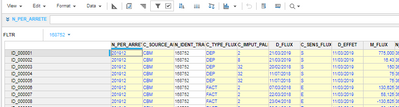•So, try the following:

• On the list in the rows, make sure you have a Top Level defined
• I am not sure which line item you are referring to, but you will need to create a line item for Min and another for Max.  For both of these, change the Summary (in the blueprint) to either Min or Max.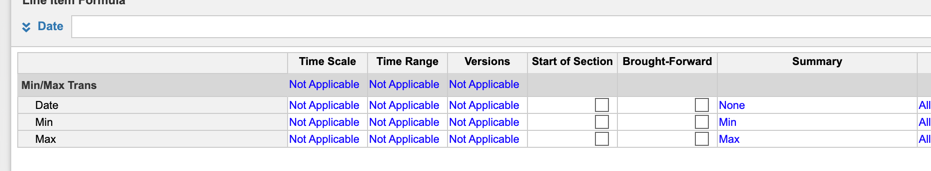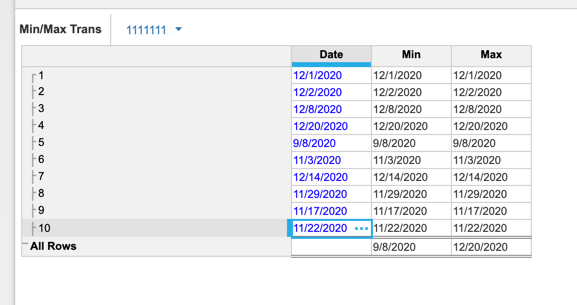Does that get you home?

Rob

•Thanks Rob,

The Line Item holding dates are the D_FLUX column.

Just to make things clearer, we are leveraging the use of IRR standard function using dates, which passes as parameters :

IRR using dates

`IRR(c, d, t, [g])`

where:

• c: Cash flow
• d: Dates
• t: Transaction List
• g: Estimate of Rate

And resolving the following equation : IRR is the iterative solution to the equation below: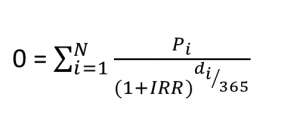• di is the number of days from the start of the first time period
• Pi is the payment in the ith period.
• N is the number of payments in and out over the entire timescale

So our challenge here is that :

1) Our first transaction isnt always holding the first time period

2) di calculated based on the "start of the first time period" seems for Anaplan engine related to the first transaction date, not the first time period among transactions

3) we may have sometimes multiples transactions occuring at the same date (which shouldnt be normally an issue when resolving the equation)

Based on upper precisions :

Would you care to elaborate on how I can leverage those min / max Line item in the IRR function itself ?

Can I use these line item as parameters for the function ?

•So, to answer your question, the MIN and MAX that I did won't help as I misunderstood your question.  And, I will also say, the IRR function is not in my wheelhouse, but I did it to work when the dates were not in order.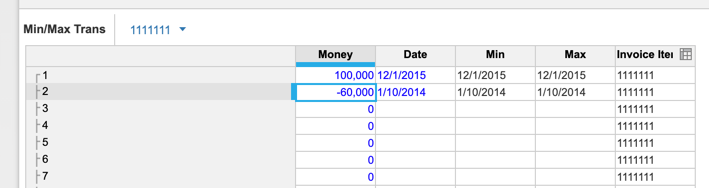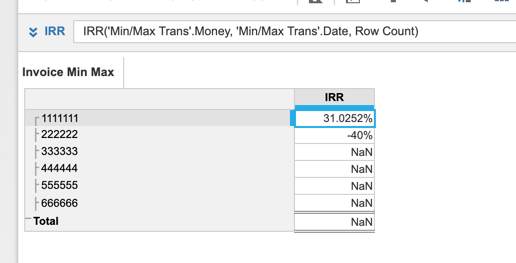Rob

•Thanks !

I think i need to look back at our issue there since dates order doesnt seem to prevent correct calculation.

Our assumption must be incorrect.

•Remember, at least one value has to be negative.